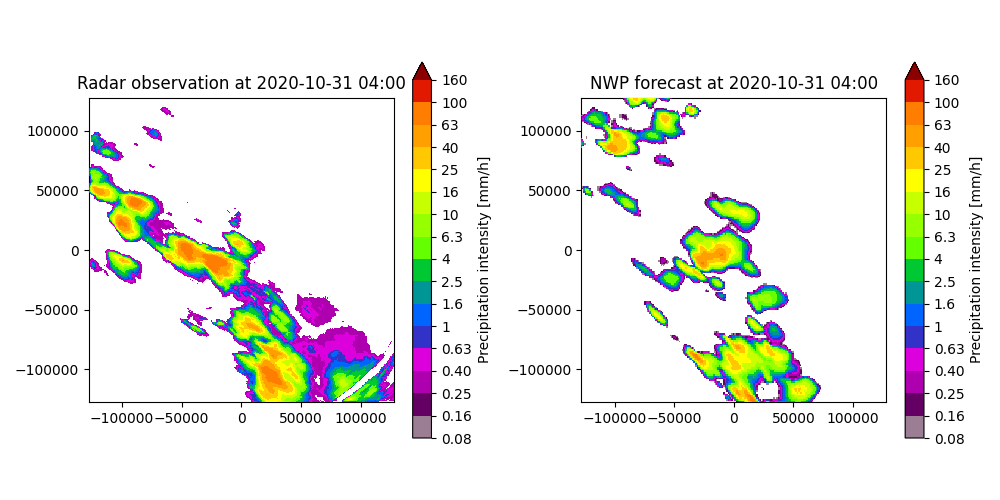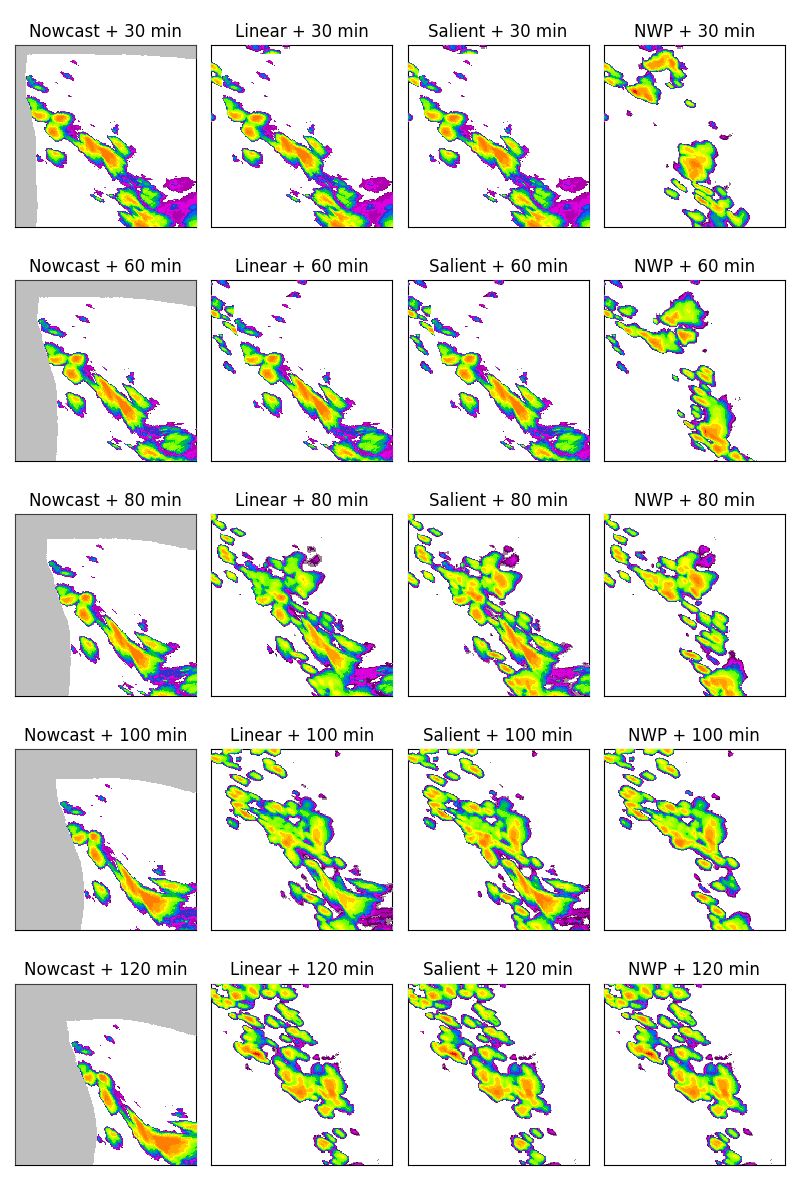# Linear blending#

This tutorial shows how to construct a simple linear blending between a STEPS ensemble nowcast and a Numerical Weather Prediction (NWP) rainfall forecast. The used datasets are from the Bureau of Meteorology, Australia.

```import os
from datetime import datetime

from matplotlib import pyplot as plt

import pysteps
from pysteps import io, rcparams, nowcasts, blending
from pysteps.utils import conversion
from pysteps.visualization import plot_precip_field
```

First, we import a sequence of 3 images of 10-minute radar composites and the corresponding NWP rainfall forecast that was available at that time.

You need the pysteps-data archive downloaded and the pystepsrc file configured with the data_source paths pointing to data folders. Additionally, the pysteps-nwp-importers plugin needs to be installed, see https://github.com/pySTEPS/pysteps-nwp-importers.

```# Selected case
# The last NWP forecast was issued at 00:00
date_nwp = datetime.strptime("202010310000", "%Y%m%d%H%M")
nwp_data_source = rcparams.data_sources["bom_nwp"]
```

### Load the data from the archive#

```root_path = radar_data_source["root_path"]
path_fmt = "prcp-c10/66/%Y/%m/%d"
fn_pattern = "66_%Y%m%d_%H%M00.prcp-c10"
timestep = 10.0

# Find the radar files in the archive
fns = io.find_by_date(
date_radar, root_path, path_fmt, fn_pattern, fn_ext, timestep, num_prev_files=2
)

importer = io.get_method(importer_name, "importer")

# Import the NWP data
filename = os.path.join(
nwp_data_source["root_path"],
datetime.strftime(date_nwp, nwp_data_source["path_fmt"]),
datetime.strftime(date_nwp, nwp_data_source["fn_pattern"])
+ "."
+ nwp_data_source["fn_ext"],
)

nwp_importer = io.get_method("bom_nwp", "importer")

# Only keep the NWP forecasts from the last radar observation time (2020-10-31 04:00)
# End of the forecast is 18 time steps (+3 hours) in advance.
precip_nwp = nwp_precip[24:43, :, :]
```
```Rainfall values are accumulated. Disaggregating by time step
```

## Pre-processing steps#

```# Make sure the units are in mm/h
converter = pysteps.utils.get_method("mm/h")

# Threshold the data
precip_nwp[precip_nwp < 0.1] = 0.0

# Plot the radar rainfall field and the first time step of the NWP forecast.
# For the initial time step (t=0), the NWP rainfall forecast is not that different
# from the observed radar rainfall, but it misses some of the locations and
# shapes of the observed rainfall fields. Therefore, the NWP rainfall forecast will
# initially get a low weight in the blending process.
plt.figure(figsize=(10, 5))
plt.subplot(121)
plot_precip_field(
)
plt.subplot(122)
plot_precip_field(
precip_nwp[0, :, :], geodata=nwp_metadata, title=f"NWP forecast at {date_str}"
)
plt.tight_layout()
plt.show()

# Only keep the NWP forecasts from 2020-10-31 04:05 onwards, because the first
# forecast lead time starts at 04:05.
precip_nwp = precip_nwp[1:]

# Transform the radar data to dB - this transformation is useful for the motion
# field estimation and the subsequent nowcasts. The NWP forecast is not
# transformed, because the linear blending code sets everything back in mm/h
# after the nowcast.
transformer = pysteps.utils.get_method("dB")
```### Determine the velocity field for the radar rainfall nowcast#

```oflow_method = pysteps.motion.get_method("lucaskanade")
```

## The linear blending of nowcast and NWP rainfall forecast#

```# Calculate the blended precipitation field
precip_blended = blending.linear_blending.forecast(
timesteps=18,
timestep=10,
precip_nwp=precip_nwp,
start_blending=60,  # in minutes (this is an arbritrary choice)
end_blending=120,  # in minutes (this is an arbritrary choice)
)
```

## The salient blending of nowcast and NWP rainfall forecast#

This method follows the saliency-based blending procedure described in [HCLK15]. The blending is based on intensities and forecast times. The blended product preserves pixel intensities with time if they are strong enough based on their ranked salience. Saliency is the property of an object to be outstanding with respect to its surroundings. The ranked salience is calculated by first determining the difference in the normalized intensity of the nowcasts and NWP. Next, the pixel intensities are ranked, in which equally comparable values receive the same ranking number.

```# Calculate the salient blended precipitation field
precip_salient_blended = blending.linear_blending.forecast(
timesteps=18,
timestep=10,
precip_nwp=precip_nwp,
start_blending=60,  # in minutes (this is an arbritrary choice)
end_blending=120,  # in minutes (this is an arbritrary choice)
saliency=True,
)
```

## Visualize the output#

Calculate the radar rainfall nowcasts for visualization

```nowcast_method_func = nowcasts.get_method("extrapolation")
precip_nowcast = nowcast_method_func(
timesteps=18,
)

# Make sure that precip_nowcast are in mm/h
```

The linear blending starts at 60 min, so during the first 60 minutes the blended forecast only consists of the extrapolation forecast (consisting of an extrapolation nowcast). Between 60 and 120 min, the NWP forecast gradually gets more weight, whereas the extrapolation forecasts gradually gets less weight. In addition, the saliency-based blending takes also the difference in pixel intensities into account, which are preserved over time if they are strong enough based on their ranked salience. Furthermore, pixels with relative low intensities get a lower weight and stay smaller in the saliency-based blending compared to linear blending. After 120 min, the blended forecast entirely consists of the NWP rainfall forecast.

```fig = plt.figure(figsize=(8, 12))

leadtimes_min = [30, 60, 80, 100, 120]

# Extrapolation
plt.subplot(n_leadtimes, 4, n * 4 + 1)
plot_precip_field(
precip_nowcast[int(leadtime / timestep) - 1, :, :],
axis="off",
colorbar=False,
)

# Nowcast with blending into NWP
plt.subplot(n_leadtimes, 4, n * 4 + 2)
plot_precip_field(
precip_blended[int(leadtime / timestep) - 1, :, :],
axis="off",
colorbar=False,
)

# Nowcast with salient blending into NWP
plt.subplot(n_leadtimes, 4, n * 4 + 3)
plot_precip_field(
precip_salient_blended[int(leadtime / timestep) - 1, :, :],
axis="off",
colorbar=False,
)

# Raw NWP forecast
plt.subplot(n_leadtimes, 4, n * 4 + 4)
plot_precip_field(
precip_nwp[int(leadtime / timestep) - 1, :, :],
axis="off",
colorbar=False,
)

plt.tight_layout()
plt.show()
```Note that the NaN values of the extrapolation forecast are replaced with NWP data in the blended forecast, even before the blending starts.

Total running time of the script: ( 0 minutes 35.038 seconds)

Gallery generated by Sphinx-Gallery